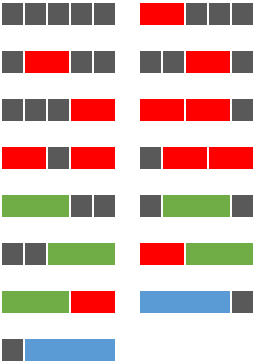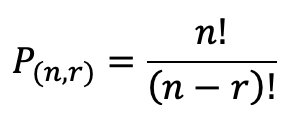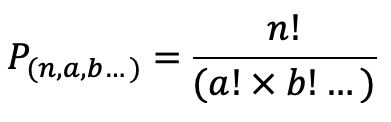## Project Euler 117I enjoy solving Project Euler problems in my downtime. Problem 117 is one that I’ve looked at multiple times, but never came up with a good solution. The premise is easy enough, but, as with most Euler problems, the scale of the problem presents issues.

Using a combination of grey square tiles and oblong tiles chosen from: red tiles (measuring two units), green tiles (measuring three units), and blue tiles (measuring four units), it is possible to tile a row measuring five units in length in exactly fifteen different ways.How many ways can a row measuring fifty units in length be tiled?

For the context of my solution, I am representing the tiles as an array or list of integers that each represent the length of a tile. For example, (1, 3, 1) would be a black, green, and black tile taking up a total of 5 spaces.

Brute Force sometimes works on these problems, but more often than not, they take far too long to complete. I don’t feel like I’ve “solved” one of these problems if the solution takes more than a second or two. My first naive attempt to solve this problem was to attack it recursively and build all the combinations of tiles. After letting it run for a few minutes, I gave up and assumed there must be a better way.

While building each tile combination was prohibitively time consuming, I found that I could still use recursion to get the base combinations for the tiles. There are only about 1000 of those, so the trick will be permuting them to get the full count. Well, as it turns out, this led to a few more problems.

Using the permutations tool provided in itertools proved to be too slow as well. First of all, it was finding too many solutions. For example:
```>>> for i in list(itertools.permutations([1, 1, 2, 2])): print i ... (1, 1, 2, 2) (1, 1, 2, 2) (1, 2, 1, 2) (1, 2, 2, 1) (1, 2, 1, 2) (1, 2, 2, 1) (1, 1, 2, 2) (1, 1, 2, 2) (1, 2, 1, 2) (1, 2, 2, 1) (1, 2, 1, 2) (1, 2, 2, 1) (2, 1, 1, 2) (2, 1, 2, 1) (2, 1, 1, 2) (2, 1, 2, 1) (2, 2, 1, 1) (2, 2, 1, 1) (2, 1, 1, 2) (2, 1, 2, 1) (2, 1, 1, 2) (2, 1, 2, 1) (2, 2, 1, 1) (2, 2, 1, 1)```
When in reality many of those are equivalent in the terms of the problem conditions. What we want is a set which looks more like this:
```>>> for i in set(itertools.permutations([1, 1, 2, 2])): print i ... (1, 1, 2, 2) (2, 1, 2, 1) (2, 1, 1, 2) (1, 2, 2, 1) (1, 2, 1, 2) (2, 2, 1, 1)```
That works, but the program still has to calculate all the permutations that we are throwing out which is time consuming; at the most, a set will have 50 tiles which is 50! permutations. That’s  30414093201713378043612608166064768844377641568960512000000000000 permutations, which is really just an insane amount! So that won’t do.

Well, we don’t need to know the permutations, just the amount of permutations! Let’s see if we can find a formula for that. The general formula that I found which researching permutations looks like this:where n is the number of terms, and r is the number of terms you are choosing from your source pool. In this case, we are going to always use all the tiles in our base combination that we want to permute, so the number of permutations is just the factorial of the number of tiles. Hmm. That doesn’t really help since it doesn’t filter out any duplicates.

Perhaps if I researched a bit more, I could have found the proper formula for this situation. Rather than read more about permutations, I decided to take one of my base combinations and do some experiments to see if I could derive a formula for this situation. I took the last combination from my list and computed the number of unique permutations in order to see what my goal should be. (This operation took over 10 minutes)
```>>> print len(set(itertools.permutations([3, 3, 4, 4, 4, 4, 4, 4, 4, 4, 4, 4, 4]))) 78```
I played around with factorials and found that for my 13 terms there would be 13!, or 6227020800, possible permutations. Since I knew I wanted the formula to result in 78, I divided the number of permutations by 78 and got 79833600. In theory, this number would be the denominator of my not-yet-existent formula. I tried grouping my 3s and 4s together; there are 2 and 11 respectively. I checked what 11! is and it turned out to be 39916800 which is exactly half of my target value for the denominator. And 2! (for the number of 3s) is 2. That seems pretty promising!My hypothesis was that multiplying the factorials of the number of similar terms together and then dividing them into the number of possible permutations would yield my desired result. I tested it out on another combination and it worked out correctly, so I modified my program to perform this operation on each combination. I ran it and checked the answer, and it was correct!And it only took .083 seconds! I’m pretty happy with the results. I’m sure a mathematician might have a more clever, direct way of solving this problem, however I think that since this solution is accurate and quick I can be proud of it. You can see the complete code on my github.

This site uses Akismet to reduce spam. Learn how your comment data is processed.